# River Network Distance Computation and Applications with `riverdist`

Package overview

Typical workflow

Limitations, notes, and caveats

Importing and displaying river network data

Basic distance calculation in a non-messy river network

Incorporating flow direction

Allowing different route-detection algorithms: a possible time-saver

Beyond individuals: summarizing or plotting at the dataset level

Editing a river network object, or fixing a messy one

Dealing with braided channels

## Package overview

The ‘riverdist’ package is intended as a free and readily-available resource for distance calculation along a river network. This package was written with fisheries research in mind, but could be applied to other fields. The ‘riverdist’ package builds upon the functionality of the ‘sp’ and ‘rgdal’ packages, which provide the utility of reading GIS shapefiles into the R environment. What ‘riverdist’ adds is the ability to treat a linear feature as a connected network, and to calculate travel routes and travel distances along that network.

## Typical workflow with ‘riverdist’

1. Import a linear shapefile using `line2network()`. The shapefile to import must be a linear feature - converting a polygon feature to a line feature will result in an outline, which will not be useable. The shapefile to import should be as simple as possible. If GIS software is available, both trimming the shapefile to the same region as the study area and performing a spatial dissolve are recommended.

2. Clean up the imported shapefile however necessary. Editing functions are provided, but the `cleanup()` function interactively steps through all the editing functions in a good order, and is recommended in nearly all cases.

3. Convert point data to river locations using `xy2segvert()` or `ptshp2segvert()`. This will snap each point to the nearest river network location.

4. Perform all desired analyses.

## Limitations, notes, and caveats

Care must be exercised in the presence of braided channels, in which multiple routes may exist between river locations. In these cases, the default route calculation provided by ‘riverdist’ will be the shortest travel route, but it is possible that the shortest travel route may not be the route of interest. Functions are provided to check for braiding, as well as select the route of interest. If no braiding is detected, route and distance calculation can switch to a more efficient algorithm.

Another important note is that only projected data can be used, both for river networks and for point data. The ‘riverdist’ environment in R does not share the ability of GIS software to project-on-the-fly, and treats all coordinates on a linear (rectangular) scale. Therefore, the projection of all data must also be the same.

## Importing and displaying river network data

Importing a river network

Displaying a river network

Checking connectedness

Converting XY data to river locations

Displaying point data in river locations

Importing a river network using `line2network()`

In a typical workflow, a user will first import a projected polyline shapefile using `line2network()`. This function reads the specified shapefile using the ‘sp’ and ‘rgdal’ packages, and adds the network connectivity, thus creating a river network object. If an unprojected shapefile is detected, an error will be generated. However, the `reproject=` argument allows the `line2network()` to re-project the shapefile before importing it as a river network.

While ‘riverdist’ does provide tools for editing a river network that do not rely on GIS software, it is strongly recommended to simplify the river shapefile as much as possible before importing into R. In particular, a spatial dissolve will likely be very helpful, if GIS software is available. This will create a few long line segments instead of many, many short segments.

``````library(riverdist)
MyRivernetwork <- line2network(path=".", layer="MyShapefile")

# Re-projecting in Alaska Albers Equal Area projection:
AKalbers <- "+proj=aea +lat_1=55 +lat_2=65 +lat_0=50 +lon_0=-154
+x_0=0 +y_0=0 +datum=NAD83 +units=m +no_defs +ellps=GRS80 +towgs84=0,0,0"

MyRivernetwork <- line2network(path=".", layer="MyShapefile", reproject=AKalbers)``````

Displaying a river network using `plot()`

Basic plotting is provided using a method of the `plot()` function. See `help(plot.rivernetwork)` for additonal plotting arguments and graphical parameters. Shown below is the Gulkana River network, included as a dataset.

``````library(riverdist)
data(Gulk)
plot(x=Gulk)``````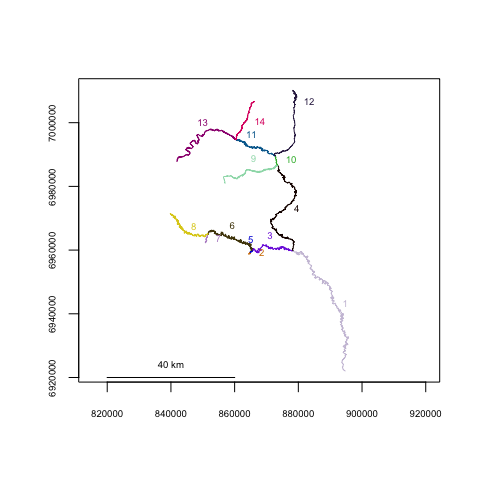Once the river network has been imported, some cleanup may be necessary, depending on the structure of the shapefile. Ideally, there should be one segment between each endpoint or junction node, and the spatial extent of the shapefile should be limited to the region or network of interest. If the user has GIS software available, it may be simplest to format the shapefile as desired before importing into R. However, functions are included for river network formatting in R if necessary. The `cleanup()` function interactively steps through the formatting functions in a good sequence, and may be a good way to get started. If there were spatial oddities with the parent shapefile (vertices out of order, or strange “jumps” in segments), the `cleanup_verts()` function interactively steps through each individual segment, providing a means to edit the vertices of each segment if needed.

``abstreams_fixed <- cleanup(abstreams0)``

Checking connectedness using `topologydots()`

For route and distance calculation to work, the topologies must be correct, with all the right segments being treated as connected. The `topologydots()` function can check for this, and plots connected segment endpoints as green, and non-connected endpoints as red. This is shown below, and all appears good.

``topologydots(rivers=Gulk)``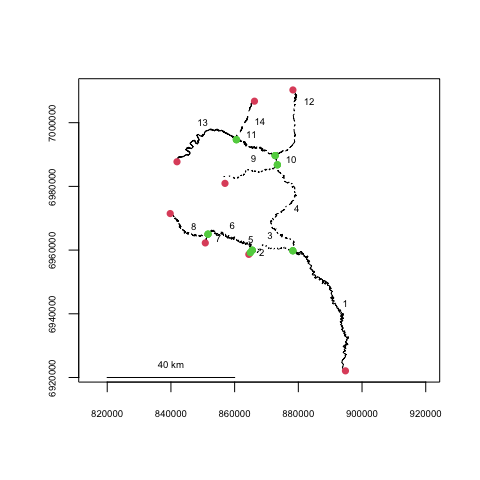Converting XY data to river locations using `xy2segvert()` and `pointshp2segvert()`

Once an appropriate river network has been obtained, point data can be read. Function `xy2segvert()` converts XY data into river locations, by “snapping” each point to the closest segment and vertex. Because of this, river locations can only be used in the context of the river network they belong to. In addition to the river locations, the snapping distance for each observation is also returned.

The `fakefish` dataset includes coordinates from a sequence of telemetry flights.

``````data(fakefish)
fakefish_riv <- xy2segvert(x=fakefish\$x, y=fakefish\$y, rivers=Gulk)
head(fakefish_riv)  # a look at the first few rows of the output``````
``````##   seg vert  snapdist
## 1   1  595 329.34419
## 2   1  399  40.27721
## 3   1  352 402.52259
## 4   1  116 525.06623
## 5   1  806 355.32753
## 6   1  505  11.34949``````
``hist(fakefish_riv\$snapdist, main="snapping distance (m)")``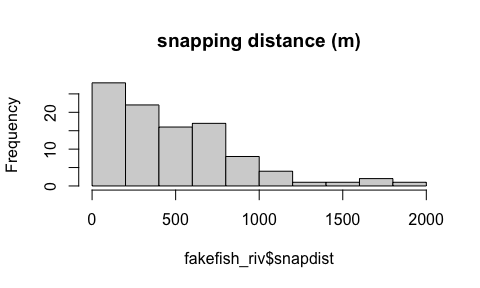Spatial data can be read directly from a point shapefile using `pointshp2segvert()`, which returns the resulting river locations added to the data table from the point shapefile.

``````segvert_from_shp <- pointshp2segvert(path=".", layer="MyPointShapefile",
rivers=MyRivernetwork)``````

Displaying point data in river locations using `riverpoints()`

The `riverpoints()` function works essentially like `points()` to overlay point data on an existing plot, but using river locations (segment and vertex). The `zoomtoseg()` function produces a plot zoomed to the specified segment number, or vector of segment numbers.

In the plot below, the raw coordinates are displayed as red circles and the river locations are displayed as blue squares.

``````zoomtoseg(seg=c(11, 14), rivers=Gulk)
points(fakefish\$x, fakefish\$y, pch=16, col="red")
riverpoints(seg=fakefish_riv\$seg, vert=fakefish_riv\$vert, rivers=Gulk, pch=15,
col="blue")``````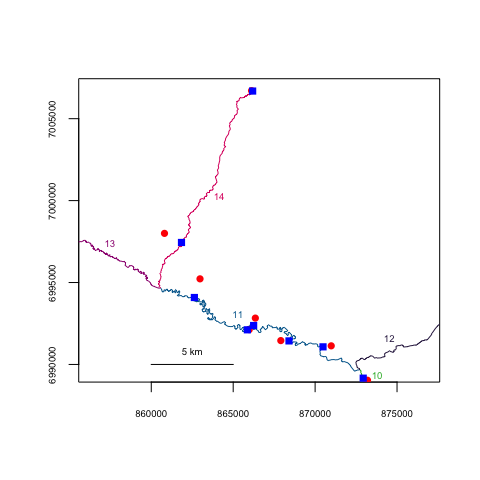## Basic distance calculation in a non-messy river network

Computing network distance

Computing network distances between sequential observations of individuals

Computing a matrix of network distances between all observations of an individual

Computing minimum observed home range for individuals

Computing a matrix of network distances between all observations

Computing a matrix of network distances between all observations of two different datasets

Computing network distance using `riverdistance()`

River network distance can be calculated directly with the `riverdistance()` function. The `riverdistance()` function calls `detectroute()` internally, which the user will probably never need, but usage is shown below. The `riverdistance()` function needs river locations (segment and vertex) for both starting and ending locations. Specifying `map=TRUE` as shown below is not necessary, but can provide a check to verify that the function is working properly.

``````# starting location: segment 7, vertex 49
# ending location: segment 14, vertex 121
detectroute(start=7, end=14, rivers=Gulk)``````
``##   7  6  3  4 10 11 14``
``riverdistance(startseg=7, startvert=49, endseg=14, endvert=121, rivers=Gulk, map=TRUE)``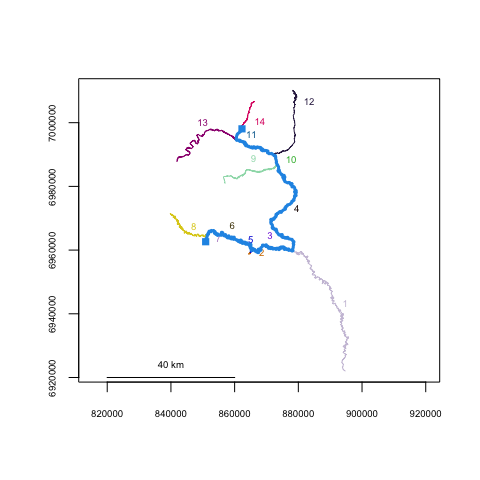``##  155435.2``

Computing network distances between sequential observations of individuals using `riverdistanceseq()`

River distance can be calculated manually between any two connected locations on a river network. However, a few common summary analyses were automated and are included in ‘riverdist’. First, the `riverdistanceseq()` function returns a matrix of the distances between sequential observations for a set of individuals observed multiple times. In the example below, three fish were observed (or not) during 5 telemetry flights. The dataset is shown first, then the output from `riverdistanceseq()`. Fish number 1 traveled 83.87 km between flights 3 and 4, and was not observed during flight 2.

``````data(smallset)
smallset``````
``````##    seg vert id flight
## 1   10   12  1      1
## 2    2   17  1      3
## 3   10   15  1      4
## 4    1  641  1      5
## 5    6  258  2      1
## 6   11  393  2      2
## 7    2   26  2      3
## 8    9  354  2      4
## 9    4  472  2      5
## 10   2   25  3      1
## 11   9  137  3      2
## 12   9  181  3      4``````
``````riverdistanceseq(unique=smallset\$id, survey=smallset\$flight, seg=smallset\$seg,
vert=smallset\$vert, rivers=Gulk)``````
``````##      1 to 2   2 to 3   3 to 4   4 to 5
## 1        NA       NA 83872.62 70277.57
## 2 127558.14 109561.1 94177.71 26741.99
## 3  86983.02       NA       NA       NA``````

Computing a matrix of network distances between all observations of an individual using `riverdistancematbysurvey()`

River distance can also be calculated between all observations of a single individual. In the output matrix shown below for individual 1, the element with row identifier `1` and column identifier `3` represents the river distance from the location observed in survey 1 to the location observed in survey 3, calculated as 83.6 km. An important note is that the distances reported are net distance between locations, and are not intended to be cumulative. Specifying `full=TRUE` reports an output matrix that includes all observations, with values of `NA` if the individual was not observed. This allows the output matrices for multiple individuals to have the same rows and columns, thus being directly comparable.

``````riverdistancematbysurvey(indiv=1, unique=smallset\$id, survey=smallset\$flight,
seg=smallset\$seg, vert=smallset\$vert, rivers=Gulk, full=FALSE)``````
``````##            1        3          4        5
## 1     0.0000 83588.99   283.6307 69993.94
## 3 83588.9911     0.00 83872.6219 45678.35
## 4   283.6307 83872.62     0.0000 70277.57
## 5 69993.9398 45678.35 70277.5705     0.00``````

Computing minimum observed home range for individuals using `homerange()`

The minimum observed (linear) home range for each individual can be calculated using `homerange()`.

``````# calculating observed minimum home range for all individuals
homerange(unique=smallset\$id, seg=smallset\$seg, vert=smallset\$vert, rivers=Gulk)``````
``````## Minumum home ranges associated with each individual
##
##   ID     range
## 1  1  99914.27
## 2  2 141136.24
## 3  3  88669.52``````

Maps can be produced by calling `plot()` on an object returned from `homerange()`. Specifying `cumulative=TRUE` will create plots with line thickness varying by the number of times an individual would have traveled a given section of river. For a cumulative plot, either a vector of survey identifiers must be used, or else the data locations must be in chronological order for each individual.

``````par(mfrow=c(1,3))
ranges <- homerange(unique=smallset\$id, survey=smallset\$flight,
seg=smallset\$seg, vert=smallset\$vert, rivers=Gulk)
plot(ranges)``````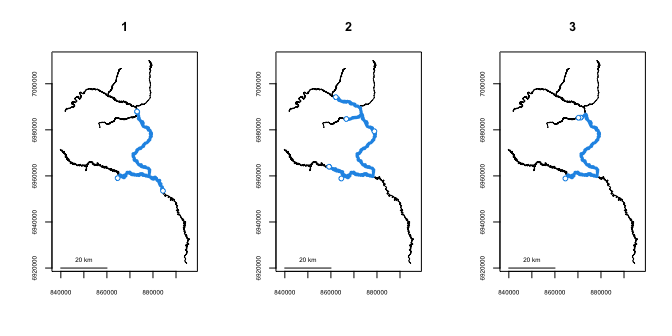``plot(ranges, cumulative=TRUE, label=TRUE, col=3)``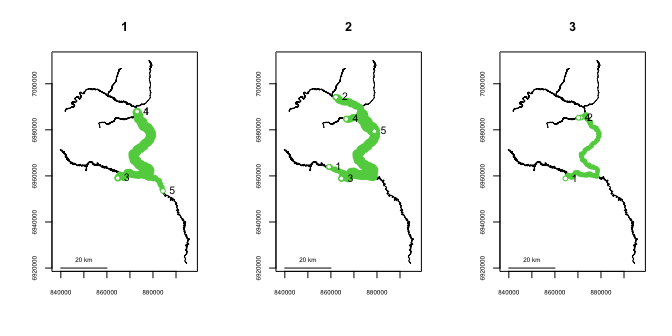The overlap between the respective home ranges of all individuals can be described using `homerangeoverlap()` This returns three matrices: first, the linear distance represented in the union of the home ranges of each pair of individuals (`\$either`), then the linear distance represented in the intersection (`\$both`), then the proportional overlap (`\$prop_both`), defined as intersection/union. Approximately 78% of the linear home range occupied by either individual 1 or 3 is shared by both of them.

The amount of overlap in home ranges can also be plotted using `plothomerangeoverlap()`.

``homerangeoverlap(ranges)``
``````## \$either
##           1        2         3
## 1  99914.27 157177.9 106065.85
## 2 157177.89 141136.2 141136.24
## 3 106065.85 141136.2  88669.52
##
## \$both
##          1         2        3
## 1 99914.27  83872.62 82517.95
## 2 83872.62 141136.24 88669.52
## 3 82517.95  88669.52 88669.52
##
## \$prop_both
##           1         2         3
## 1 1.0000000 0.5336159 0.7779879
## 2 0.5336159 1.0000000 0.6282548
## 3 0.7779879 0.6282548 1.0000000``````
``plothomerangeoverlap(ranges)``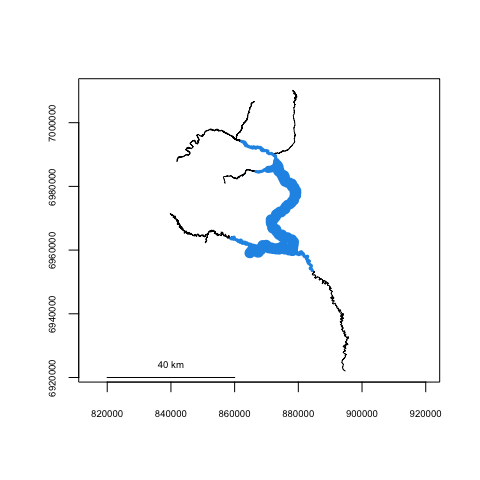Computing a matrix of network distances between all observations using `riverdistancemat()`

A matrix of the river network distance between every observation and every other observation can also be calculated using `riverdistancemat()`. A use for this function might be if the user wishes to calculate all distances at once, and subset later.

``````dmat <- riverdistancemat(smallset\$seg,smallset\$vert,Gulk)
round(dmat)[1:7,1:7]  # only showing the first 7 rows & columns for clarity``````
``````##        1      2      3     4      5      6      7
## 1      0  83589    284 69994 101953  25605  83956
## 2  83589      0  83873 45678  22200 109194    367
## 3    284  83873      0 70278 102236  25322  84239
## 4  69994  45678  70278     0  64042  95599  46045
## 5 101953  22200 102236 64042      0 127558  22567
## 6  25605 109194  25322 95599 127558      0 109561
## 7  83956    367  84239 46045  22567 109561      0``````

The `logical` argument can be used for subsetting, if the full network distance matrix is not needed. This capability is shown below, calculating the distance matrix only for observations occurring on segment number 2. The `ID` argument can be used with a vector of observation labels, to display row and column labels that may be easier to interpret than observation indices.

``````logi1 <- (smallset\$seg==2)

# constructing observation labels
obsID <- paste0("id",smallset\$id,"-flight",smallset\$flight)

riverdistancemat(seg=smallset\$seg, vert=smallset\$vert, rivers=Gulk, logical=logi1,
ID=obsID)``````
``````##             id1-flight3 id2-flight3 id3-flight1
## id1-flight3      0.0000   366.62343   326.28884
## id2-flight3    366.6234     0.00000    40.33459
## id3-flight1    326.2888    40.33459     0.00000``````

Computing a matrix of network distances between all observations of two different datasets using `riverdistancetofrom()`

A matrix of river network distance between two location datasets can be calculated using `riverdistancetofrom()`, which can be similarly subsetted using the `logical1` and `logical2` arguments. Row and column names can be added using the `ID1` and `ID2` arguments.

An example of the use of this function might be computation of distance between observations of instrumented fish and a set of fixed river locations, such as contaminant sites. In this case, a similar function `upstreamtofrom()` (discussed later) might be used, which would give the means to calculate up- or downstream distance from contaminant sites, with the option of examining flow-connectedness.

``````streamlocs.seg <- c(2,2,2)
streamlocs.vert <- c(10,20,30)
streamlocs.ID <- c("loc A","loc B","loc C")

logi2 <- (smallset\$seg==2)
obsID <- paste0("id",smallset\$id,"-flight",smallset\$flight)

riverdistancetofrom(seg1=streamlocs.seg, vert1=streamlocs.vert, seg2=smallset\$seg,
vert2=smallset\$vert, ID1=streamlocs.ID, ID2=obsID, logical2=logi2,
rivers=Gulk)``````
``````##       id1-flight3 id2-flight3 id3-flight1
## loc A   129.95819    496.5816    456.2470
## loc B    99.42056    267.2029    226.8683
## loc C   479.65380    113.0304    153.3650``````

## Incorporating flow direction

Defining flow direction of a river network

Calculating flow direction and directional network distance

Automations of flow direction and directional network distance

Defining flow direction of a river network using `setmouth()`

Flow direction and directional (upstream) distance can also be calculated. For this to be accomplished, the segment and vertex of the river “mouth”, or lowest point must first be identified. The segment containing the river mouth can be visually identified from a plot of the river network. In the Gulkana River example, the lowest segment happens to be segment 1. Identifying the lowest vertex of segment 1 can be done using the plot produced by `showends()`, shown below. In this case, the mouth vertex happens to be vertex 1. This will not necessarily be the case. After importing a shapefile into R, the segment vertices will be stored in sequential order, but not necessarily by flow direction. Specifying the segment and vertex coordinates of the river network mouth can be done using `setmouth()` as shown below, though it can also be set manually by direct assignment.

``showends(seg=1,rivers=Gulk)``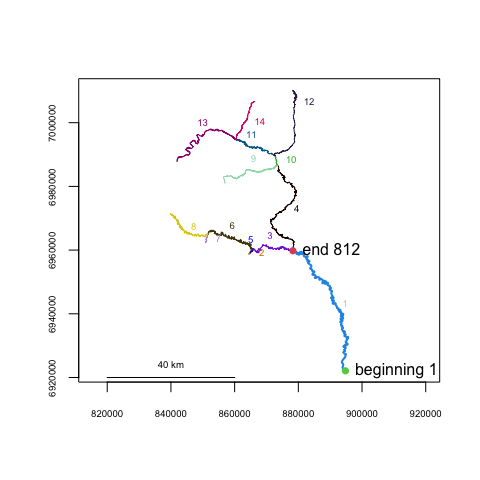``Gulk1 <- setmouth(seg=1, vert=1, rivers=Gulk)``

Calculating flow direction using `riverdirection()` and directional network distance using `upstream()`

If the flow direction has been established by specifying the river network mouth, river direction can be calculated using `riverdirection()`, and upstream distance can be calculated using `upstream()`. If the input river locations are flow-connected, `riverdirection()` returns “up” if the second location is upstream of the first and “down” if downstream. In the flow-connected case, `upstream()` returns the network distance as positive if the second location is upstream of the first, and negative if downstream. If the input locations are not flow-connected, `riverdirection()` returns “up” if the total upstream distance is greater than the total downstream distance, and “down” otherwise. In the non flow-connected case, upstream distance in `upstream()` can return one of two things, depending on the user’s research intent. Specifying `net=TRUE` will return the “net” distance (upstream distance - downstream distance between the two locations). Specifying `net=FALSE` (the default) will return the total distance between the two locations, with the sign depending on whether the upstream distance exceeds the downstream distance.

For example, the route between two points goes downstream along a river for 100m, then up 20m on a tributary. Specifying `net=TRUE` will return a distance of -80m. Specifying `net=FALSE` will return a distance of -120m.

Specifying `flowconnected=TRUE` in both `riverdirection()` and `upstream()` will only return distances or directions if the input locations are flow-connected, and will return `NA` otherwise.

``````zoomtoseg(seg=c(6,3), rivers=Gulk)
riverpoints(seg=c(6,4), vert=c(250,250), col=4, pch=15, rivers=Gulk1)
#riverdistance(startseg=6, endseg=4, startvert=250, endvert=250, rivers=Gulk1, map=TRUE)
text(c(859122.4, 872104.1), c(6964127.4,6969741.0), pos=c(3, 4),
labels=c("beginning", "end"))``````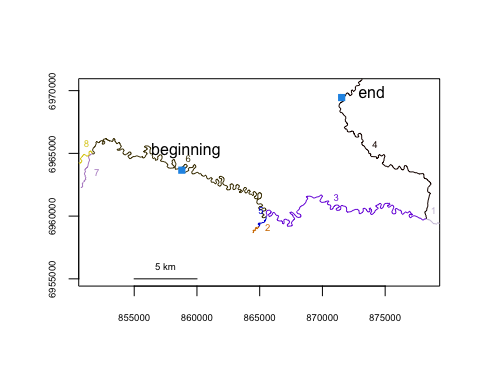``riverdirection(startseg=6, endseg=4, startvert=250, endvert=250, rivers=Gulk1)``
``##  "down"``
``upstream(startseg=6, endseg=4, startvert=250, endvert=250, rivers=Gulk1, net=FALSE)``
``##  -66235.32``
``upstream(startseg=6, endseg=4, startvert=250, endvert=250, rivers=Gulk1, net=TRUE)``
``##  -28636.75``
``````upstream(startseg=6, endseg=4, startvert=250, endvert=250, rivers=Gulk1,
flowconnected=TRUE)``````
``##  NA``

Automations of flow direction and directional network distance

River direction and upstream distance are also applied in functions `riverdirectionseq()` and `upstreamseq()` which work like `riverdistanceseq()`, `riverdirectionmatbysurvey()` and `upstreammatbysurvey()` which work like `riverdistancematbysurvey()`, `riverdirectionmat()` and `upstreammat()` which work like `riverdistancemat()`, and `riverdirectiontofrom` and `upstreamtofrom` which work like `riverdistancetofrom`. The `upstreamseq()`, `upstreammatbysurvey()`, and `upstreammat()` use the additional `net=` argument, and all river direction and upstream distance functions use the additional `flowconnected=` argument.

## Allowing different route-detection algorithms: a possible time-saver

Currently three algorithms are implemented to detect routes between river locations, with the functions automatically selecting the most appropriate unless another is specified. Dijkstra’s algorithm is used by default (`algorithm="Dijkstra"`), which returns the shortest route in the presence of braiding. The sequential algorithm (`algorithm="sequential"`) may be used, which returns the first complete route detected. The sequential algorithm is much slower and is not recommended in nearly all cases, but is retained as an option for certain checks.

If many distance calculations or a more sophisticated analysis is to be conducted, it is highly recommended to run `buildsegroutes()`, allowing `algorithm="segroutes"` to be used. This adds route and distance information to the river network object, greatly simplifying distance calculation, and reducing processing time for each distance calculation. The `buildsegroutes()` function also includes an option to calculate a distance lookup table as well. This may take a few seconds to run, but will reduce computation times even further - cutting the already-fast segment route algorithm time by 50-80%. Lookup tables can be calculated directly using `buildlookup()`, but may be extremely slow to calculate without running `buildsegroutes()` first.

In the example below, distance is calculated between the two points in a complex river network, using all three route detection algorithms, and calculating the time requirement for a single calculation.

``````data(abstreams)

tstart <- Sys.time()
riverdistance(startseg=120, startvert=10, endseg=131, endvert=10, rivers=abstreams,
algorithm="sequential")``````
``##  68937.76``
``Sys.time()- tstart``
``## Time difference of 0.2085481 secs``
``````tstart <- Sys.time()
riverdistance(startseg=120, startvert=10, endseg=131, endvert=10, rivers=abstreams,
algorithm="Dijkstra")``````
``##  68937.76``
``Sys.time()- tstart``
``## Time difference of 0.004094839 secs``
``````tstart <- Sys.time()
riverdistance(startseg=120, startvert=10, endseg=131, endvert=10, rivers=abstreams)``````
``##  68937.76``
``````# Note: it is not necessary to specify the algorithm here: the distance function
# will automatically select the fastest algorithm unless otherwise specified.
Sys.time()- tstart``````
``## Time difference of 0.001580954 secs``

In this case, building segment routes and distance lookup tables takes a little more than a second, even with a fairly complex river network. Calculating a single distance afterwards takes about 80 microseconds using segment routes and about 25 microseconds using the lookup tables. This dramatically saves time if multiple distances are to be computed, such as in a large matrix of distances, or multiple analyses. Kernel density and K-function analysis is probably prohibitively slow otherwise.

## Beyond individuals: summarizing or plotting at the dataset level

Calculating and plotting kernel density

Spatial data spread for each flight event

Plotting clustering or dispersal using K-functions

Summarizing up-river position

Plotting a summary matrix or distance sequence

Plotting all upstream movements

Calculating and plotting kernel density using `riverdensity()` and `plotriverdensity()`

A method has been provided to display kernel density calculated from point data, using river network distance. The `makeriverdensity()` function calculates scaled kernel density at approximately regularly-spaced river network locations, with the linear resolution specified by the optional `resolution=` argument. A gaussian (normal) kernel is used by default, but a rectangular (simple density) kernel can be used as well. The `survey=` argument may also be used with a vector of survey identifiers corresponding to the point data. If the `survey=` argument is used, `makeriverdensity()` will calculate separate densities for each unique survey, and a method of `plot()` will produce a separate plot for each unique survey.

The `plot()` function method can display densities using line thickness, color, or both. For additional plotting arguments including possible color ramps, see `?plot.riverdensity`.

Densities for nine of the ten Fakefish surveys are shown below.

``````data(Gulk, fakefish)
fakefish_density <- makeriverdensity(seg=fakefish\$seg, vert=fakefish\$vert, rivers=Gulk,
survey=fakefish\$flight.date, resolution=2000, bw=10000)
par(mfrow=c(3,3))
plot(x=fakefish_density, ramp="blue", dark=0.85, maxlwd=15,
whichplots=c(1:8,10))   # showing only nine plots for clarity``````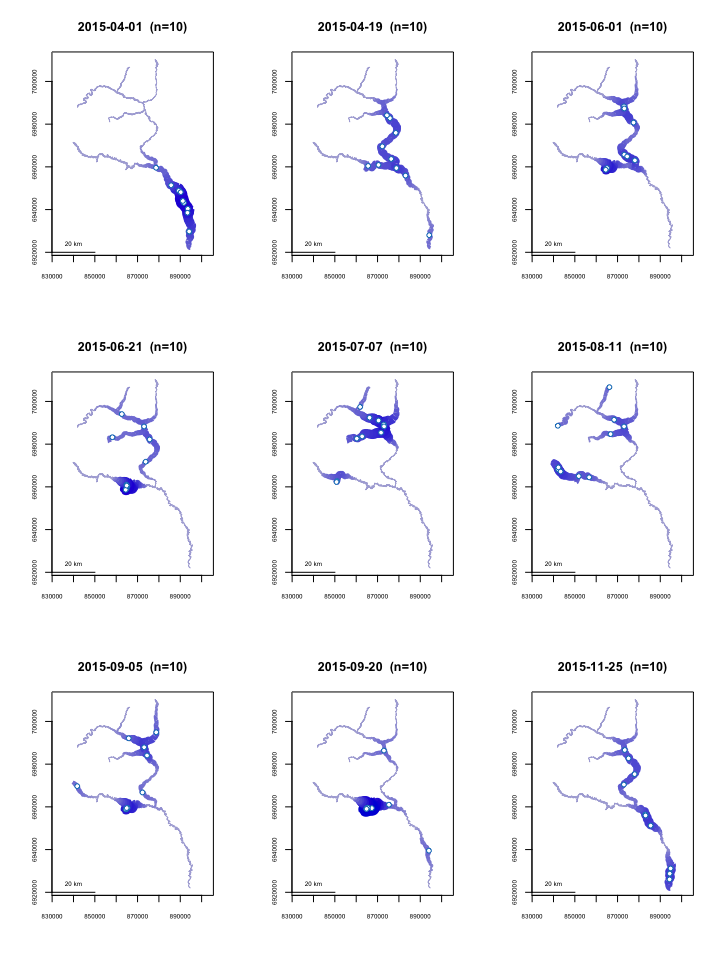An alternate use of `homerange()` to give the spatial data spread for each flight event

The `homerange()` function could also be used to give a measure of the spatial spread of observations for each flight event, by using the flight identifier instead of individual identifier in the `unique=` argument.

In this case, the observations are the most closely spaced in the first flight event, and the most widely spaced in the August 11 flight event. Interestingly, the density plot of the August 11 flight shows many of the the individuals to be located near one another, but spread to multiple tributaries, with little presence in between. Considering this event to be the largest spread may still be appropriate, depending on the study, since all individuals were observed as concentrated in the lower mainstem on April 1.

``````x <- homerange(unique=fakefish\$flight.date, seg=fakefish\$seg, vert=fakefish\$vert, rivers=Gulk)
x``````
``````## Minumum home ranges associated with each individual
##
##            ID     range
## 1  2015-04-01  66026.33
## 2  2015-04-19 145231.00
## 3  2015-06-01  84856.73
## 4  2015-06-21 136195.56
## 5  2015-07-07 176480.46
## 6  2015-08-11 235446.27
## 7  2015-09-05 171769.53
## 8  2015-09-20 130524.91
## 9  2015-10-29 140431.19
## 10 2015-11-25 124827.73``````
``````plot(x\$range, type='b', xaxt='n', xlab="", ylab="range (m)", cex.axis=.6)
axis(1,at=1:10,labels=sort(unique(fakefish\$flight.date)), cex.axis=.6, las=3)``````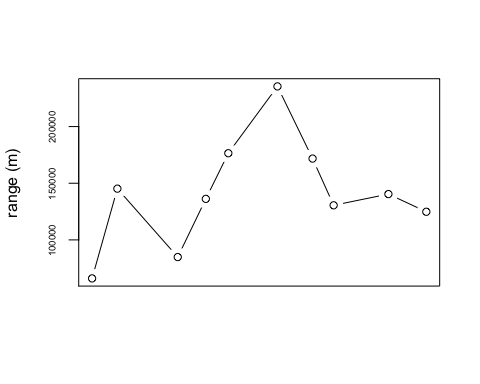Plotting clustering or dispersal using K-functions, with `kfunc()`

K-functions, defined here as the average proportion of additional individuals located within a given distance of each individual, can be a useful tool for investigating evidence of clustering or dispersal. Calling `kfunc()` and specifying the flight indentifier will result in a sequence of plots, each displaying the K-function associated with each survey.

Unless otherwise specified, each plot will be overlayed with a confidence envelope, calculated by resampling all within-survey distances, thus creating a null distribution under the assumption that clustering is independent of survey. Thus, a K-function above the envelope at a small distance range can be seen as evidence that locations were more clustered than expected for that survey; conversely, a K-function below the envelope at a small distance range can be seen as evidence that locations were more dispersed than expected for that survey.

The plots below show relatively high amounts of clustering for the 2015-04-01 and 2015-09-20 surveys. The 2015-07-07 and especially 2015-08-11 surveys show possible clustering at small distances and very strong dispersal at medium distances, which can be corroborated with the density maps shown above..

``````par(mfrow=c(3,3))
kfunc(seg=fakefish\$seg, vert=fakefish\$vert, rivers=Gulk, survey=fakefish\$flight.date,
maxdist=200000, whichplots=c(1:8,10))   # showing only nine plots for clarity``````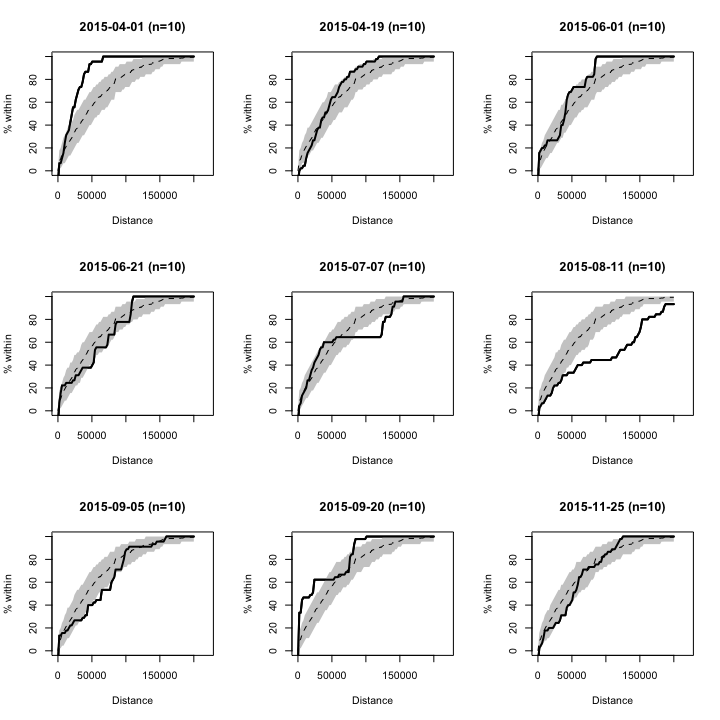Summarizing up-river position, defined as distance from mouth, using `mouthdist()` for one observation and `mouthdistbysurvey()` for a dataset

In some cases, a meaningful summary measure may be the up-river position of each observation. Distance between an individual observation and the river mouth can be calculated using `mouthdist()`. In the case of multiple observations of a set of individuals, a summary matrix can be calculated using `mouthdistbysurvey()`, which returns a matrix of distances from the river mouth, with each row corresponding to a unique individual and each column corresponding to a unique survey.

``mouthdist(seg=fakefish\$seg, vert=fakefish\$vert, rivers=Gulk)``
``##  56016.35``
``````x <- mouthdistbysurvey(unique=fakefish\$fish.id, survey=fakefish\$flight,
seg=fakefish\$seg, vert=fakefish\$vert, rivers=Gulk)

round(x)``````
``````##        1      2      3      4      5      6      7      8      9     10
## 1  26535 121619     NA 131182 162899     NA     NA 105966 101516  65373
## 2  10333     NA     NA 105166     NA     NA     NA  99972     NA     NA
## 3     NA   7581 106455     NA 150986 164013 125509 106237 102427  14046
## 4     NA 108482 117334     NA 145338 131182 146021     NA     NA   4878
## 6     NA 125610     NA     NA     NA 165423 105344     NA 108398     NA
## 7  10153  75961  88672 155589 131218     NA     NA     NA     NA     NA
## 8  56016     NA 106901     NA     NA     NA 166606 104745  95707  64041
## 9  46998  96425 130164 106331     NA     NA     NA 105344     NA 122739
## 10 36393     NA     NA  99958 132170     NA     NA  82537  75916     NA
## 11 76180     NA     NA     NA     NA 139313     NA 104850  11434 107265
## 12    NA  63984     NA 106901     NA     NA 130796     NA     NA  97886
## 13    NA     NA     NA 120679 145370 174795 142849     NA     NA     NA
## 14 31502     NA  80465 105835 144800 140778 106050     NA 126070  56293
## 15    NA  92050 131218     NA 147101 130009     NA     NA  52751     NA
## 16 45619     NA     NA     NA     NA     NA 106656 104879 117856   8714
## 17 38397     NA 105165     NA 133124 160212     NA     NA     NA     NA
## 18    NA  83037  86457 153561     NA     NA  90940     NA     NA 129706
## 19    NA 103833     NA 107267     NA 196411     NA 130493  89983     NA
## 20    NA     NA 106260     NA 136400 141994 104879  29574     NA     NA``````

Plotting the summary matrix from `mouthdistbysurvey()` or other distance sequence using `plotseq()`

The resulting matrix of up-river position returned from `mouthdistbysurvey()`, or another distance sequence returned from `riverdistanceseq()` or `upstreamseq()`, can be plotted using `plotseq()`. A few types of plots are available, with two shown below. Additional `type=` arguments can be seen by calling `?plotseq`.

The user is cautioned to use any plots returned as descriptive tools only, as ANOVA-type inference would likely be inappropriate without accounting for repeated-measures and/or serial autocorrelation.

In the example below, the plot shows evidence of the instrumented fish beginning the sampling period near the river mouth, then migrating upriver, and finally ending the sampling period near the mouth.

``````x <- mouthdistbysurvey(unique=fakefish\$fish.id, survey=fakefish\$flight.date,
seg=fakefish\$seg, vert=fakefish\$vert, rivers=Gulk)

par(mfrow=c(1,2))
plotseq(seqbysurvey=x)
plotseq(seqbysurvey=x, type="dotline")``````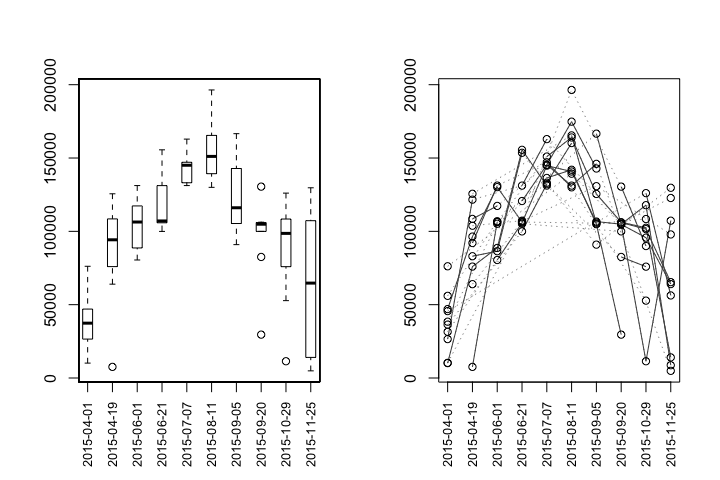``par(mfrow=c(1,1))``

Depending on the study, `plotseq()` may be useful in plotting the upstream distance between observations of all individual, as calculated in `upstreamseq()`. It is worth noting that the outputs from both `upstreamseq()` and `riverdistanceseq()` may have many empty cells, if individuals were missed on any flights. For the purpose of clarity, the default plot type is used below, which produces boxplots. However, in this case, a jittered dotplot may be more appropriate due to the small sample sizes, and can be produced by specifying `type="dotplot"`.

In this case, the plots show evidence that movement was generally up-river between flights 1 and 2 and between flights 2 and 3, that there was the greatest variability in upstream movement between flights 5 and 6, and that movement was generally down-river in the latter part of the study.

``````x <- upstreamseq(unique=fakefish\$fish.id, survey=fakefish\$flight, seg=fakefish\$seg,
vert=fakefish\$vert, rivers=Gulk)
round(x)``````
``````##    1 to 2 2 to 3 3 to 4 4 to 5  5 to 6  6 to 7 7 to 8 8 to 9 9 to 10
## 1   95084     NA     NA  31717      NA      NA     NA -54220  -36143
## 3      NA  98874     NA     NA  161737 -136261 -78484  -3810  -88381
## 4      NA   8852     NA     NA  -14156   14839     NA     NA      NA
## 6      NA     NA     NA     NA      NA  -62068     NA     NA      NA
## 7   65807  12711  66918 -24372      NA      NA     NA     NA      NA
## 8      NA     NA     NA     NA      NA      NA -62652  -9038  -31666
## 9   49427  33739 -83233     NA      NA      NA     NA     NA      NA
## 10     NA     NA     NA  32212      NA      NA     NA  -6621      NA
## 11     NA     NA     NA     NA      NA      NA     NA -93416   95831
## 13     NA     NA     NA 112787  166903  -52141     NA     NA      NA
## 14     NA     NA  33038  41936 -132316  -93566     NA     NA  -69777
## 15     NA  70005     NA     NA -123847      NA     NA     NA      NA
## 16     NA     NA     NA     NA      NA      NA  -1777  69473 -109142
## 17     NA     NA     NA     NA  140074      NA     NA     NA      NA
## 18     NA   3419  67104     NA      NA      NA     NA     NA      NA
## 19     NA     NA     NA     NA      NA      NA     NA -67214      NA
## 20     NA     NA     NA     NA  125132  -38174 -75305     NA      NA``````
``````par(mfrow=c(1,2))
plotseq(seqbysurvey=x)

plotseq(seqbysurvey=x, type="dotplot")
abline(h=0, lty=3)``````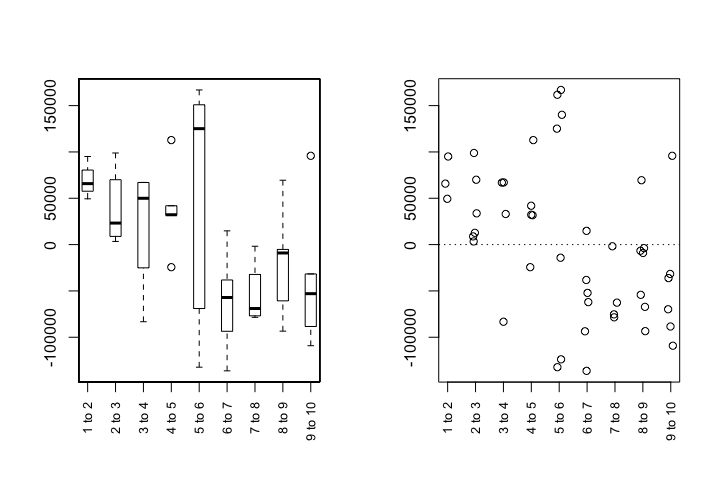Plotting all upstream movements using `matbysurveylist()` and `plotmatbysurveylist()`

In a more generalized application, the `matbysurveylist()` function calculates the distances or upstream distances, between each pair of observation events, for all individuals. Functionally, this is a summary of the outputs of `riverdistancematbysurvey()` or `upstreammatbysurvey()`, but for all individuals in a dataset. The output from `matbysurveylist()` can then be plotted using `plotmatbysurveylist()`, providing a one-plot summary of all movements within a dataset. It is recommended to use the default `method="upstream"` in `matbysurveylist()`, which calculates directional (upstream) distances, which will result in a more informative plot.

The output plot is the upper triangle of a matrix of plots, in which plot `[i,j]` represents the upstream distances traveled between observation `i` and observation `j`, for all individuals observed in those observations. Each plot is overlayed with a horizontal line at an upstream distance of zero, for an illustration of up-river or down-river movement trend for that pairwise movement. It is worth noting that all sequential pairings of events (first to second, second to third, etc.) fall on the lower edge of the triangle, and this sequence of plots is the same as those given by `plotseq()` in the previous example.

Three types of plots can be produced using the `type=` argument. The default boxplot type (`type="boxplot"`) is shown below. Alternately, if the `type=` argument is set to `"confint"`, each plot gives a line showing the extent of an approximate 95% confidence interval for the mean upstream distance traveled. Inference should be made with caution, as sample sizes are likely to be small, and no attempt has been made at using a family-wise confidence level. However, plotting confidence intervals may be further illustrative of trend. A jittered dotplot may be produced by specifying `type="dotplot"`, which will be the most appropriate in the case of small sample sizes. The default boxplot is shown below for the purpose of clarity, but a jittered dotplot would likely be a better choice in this instance.

``````matbysurveylist <- matbysurveylist(unique=fakefish\$fish.id, survey=fakefish\$flight, seg=fakefish\$seg,
vert=fakefish\$vert, rivers=Gulk)
plotmatbysurveylist(matbysurveylist)``````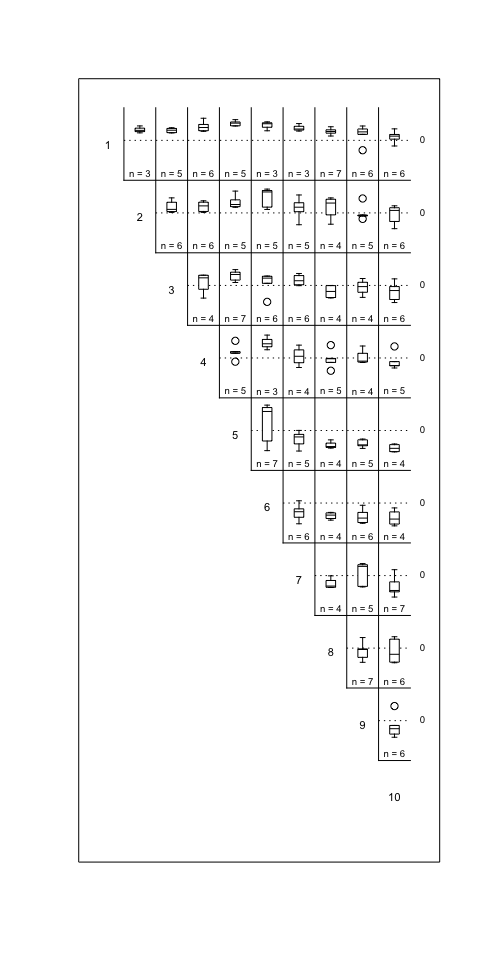## Editing a river network object, or fixing a messy one

All-purpose river network cleanup

Issue: the river network contains unneeded or unconnected segments

Issue: the river network contains sequential “runs” of segments that do not otherwise branch

Issue: the river network segments do not break where they should

Issue: segments that should connect do not

Issue: the river network contains segments that are smaller than the connectivity tolerance

Issue: A long straight-line section of the river network does not contain vertices between endpoints

Ideally, the shapefile used to define a river network object should be refined in GIS before importing into R. That being said, there are sure to be instances in which it is advantageous or necessary to make changes to the river network object within R.

All-purpose river network cleanup using `cleanup()`

In many cases, the `cleanup()` function will be the recommended first step in fixing a messy river network object after importing it. The `cleanup()` function should be called within the console, and interactively calls the editing functions in sequence. It then returns a new, edited river network object which can be edited further, or used as is. It may even be the most straightforward to call `cleanup()` multiple times. If there were spatial oddities with the parent shapefile (vertices out of order, or strange “jumps” in segments), the `cleanup_verts()` function interactively steps through each individual segment, providing a means to edit the vertices of each segment if needed.

Usage will look like

``````data(abstreams0)  # a messy river network
abstreams_fixed <- cleanup(abstreams0)  # fixing many problems``````

Issue: the river network contains unneeded or unconnected segments - fixes using `trimriver()`, `trimtopoints()`, and `removeunconnected()`

This is a very likely issue, particularly if the network was imported without changes in a GIS environment. River network segments can be manually removed using `trimriver()`, as shown below. Using the argument `trim=` removes the specified segments, and using the argument `trimto=` removes all but the specified segments. The example below is fairly simplistic, but illustrates the usage of the `trim=` and `trimto=` arguments.

``````Gulk_trim1 <- trimriver(trim=c(10,11,12,13,14), rivers=Gulk)
Gulk_trim2 <- trimriver(trimto=c(10,11,12,13,14), rivers=Gulk)

par(mfrow=c(1,3))
plot(x=Gulk, main="original")
plot(x=Gulk_trim1, main="trim=c(10,11,12,13,14)")
plot(x=Gulk_trim2, main="trimto=c(10,11,12,13,14)")``````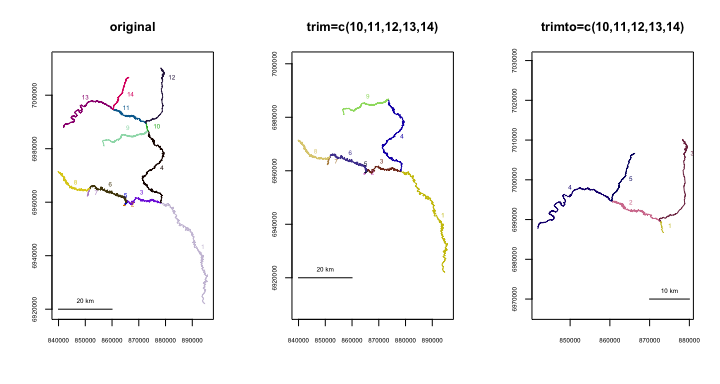It is also possible to trim a river network to include only segments that are within a spatial tolerance of a set of X-Y points. The `trimtopoints()` function offers three methods of doing this. Specifying `method="snap"` (the default) returns a river network made up only of the closest segments to the input points. This is the simplest method, but may result in spatial gaps, as shown in the example below. Specifying `method="snaproute"` returns a network of the closest segments to the input points, but also includes any segments necessary to maintain a connected network. Specifying `method="buffer"` returns a river network made up of segments with endpoints or midpoints located within a specified “buffer” distance of the input points. This may be advantageous if the user wants to include segments that are near, but not directly proximal, to the input points.

``````data(Kenai3)
x <- c(174185, 172304, 173803, 176013)
y <- c(1173471, 1173345, 1163638, 1164801)
Kenai3.buf1 <- trimtopoints(x=x, y=y, rivers=Kenai3, method="snap")
Kenai3.buf2 <- trimtopoints(x=x, y=y, rivers=Kenai3, method="snaproute")
Kenai3.buf3 <- trimtopoints(x=x, y=y, rivers=Kenai3, method="buffer", dist=5000)

plot(x=Kenai3, main="original")
points(x, y, pch=15, col=4)
legend(par("usr"), par("usr"), legend="points to buffer around", pch=15,
col=4, cex=.6)``````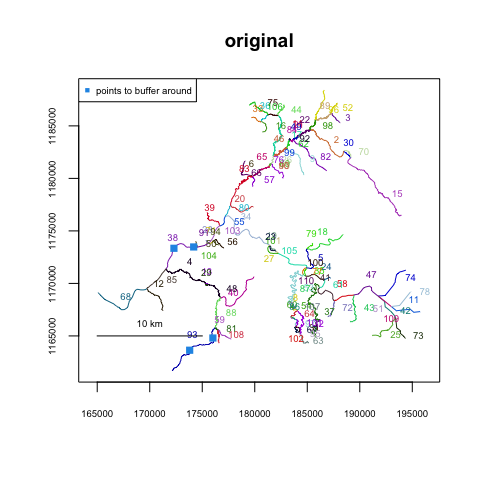``````par(mfrow=c(1,3))
plot(x=Kenai3.buf1, main="snap")
points(x, y, pch=15, col=4)
plot(x=Kenai3.buf2, main="snaproute")
points(x, y, pch=15, col=4)
plot(x=Kenai3.buf3, main="buffer, dist=5000")
points(x, y, pch=15, col=4)``````